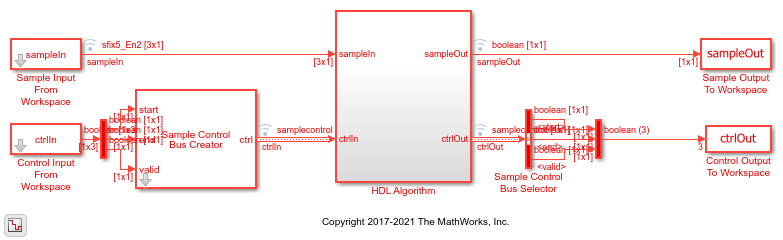# Turbo Decode Streaming Samples

This example shows how to use the LTE Turbo Decoder block to decode data, and how to compare the hardware-friendly design with the results from LTE Toolbox™.

1. Generate frames of random input samples in MATLAB®. Encode the samples and add noise to the data.

2. Decode the data using the LTE Toolbox function, `lteTurboDecode`.

3. Convert framed input data to a stream of samples and import the stream into Simulink®.

4. To decode the samples using a hardware-friendly architecture, execute the Simulink model, which contains the LTE Turbo Decoder block.

5. Export the stream of decoded bits to the MATLAB workspace.

6. Convert the sample stream back to framed data, and compare the frames with the decoded frames from Step 2.

Generate input data frames. Turbo encode the data, modulate the message, and add noise to the resulting constellation. Demodulate the noisy constellation and generate soft bit values. Generate reference decoded data using `lteTurboDecode`. For the hardware-friendly model, convert the soft bits into a fixed-point data type.

```rng(0); numframes = 2; txBits = cell(1,numframes); softBits = cell(1,numframes); rxBits = cell(1,numframes); inframes = cell(1,numframes); for ii = 1:numframes txBits{ii} = randi([0 1],6144,1); codedData = lteTurboEncode(txBits{ii}); txSymbols = lteSymbolModulate(codedData,'QPSK'); noise = 0.5*complex(randn(size(txSymbols)),randn(size(txSymbols))); rxSymbols = txSymbols + noise; softBits{ii} = lteSymbolDemodulate(rxSymbols,'QPSK','Soft'); rxBits{ii} = lteTurboDecode(softBits{ii}); inframes{ii} = fi(softBits{ii},1,5,2); end ```

Serialize input data for the Simulink model. Leave enough time between frames for each frame to be fully decoded before the next one starts. The LTE Turbo Decoder block takes 2 * `numTurboIterations` * HalfIterationLatency + ( `inframesize` / `samplesizeIn` ) cycles to complete decoding of a frame. For details of the HalfIterationLatency calculation see the Turbo Decoder block reference page.

The LTE Turbo Decoder block expects input samples are interleaved with the parity bits.

Hardware-friendly input: `S_1 P1_1 P2_1 S2 P1_2 P2_2 ... Sn P1_n P2_n`

LTE Toolbox input: `S_1 S_2 ... S_n P1_1 P1_2 ... P1_n P2_1 P2_2 ... P2_n`

Reorder the samples using the interleave option of the `whdlFramesToSamples` function.

```inframesize = size(inframes{1},1); %includes 4 tail bit samples encoderrate = 3; % rate 1/3 Turbo code samplesizeIn = encoderrate; % 3 samples in at a time idlecyclesbetweensamples = 0; outframesize = size(txBits{1},1); numTurboIterations = 6; halfIterationLatency = (ceil(outframesize/32)+3)*32; % window size=32 algframedelay = 2*numTurboIterations*halfIterationLatency+(inframesize/samplesizeIn); idlecyclesbetweenframes = algframedelay; interleaveSamples = true; [sampleIn,ctrlIn] = ... whdlFramesToSamples(inframes, ... idlecyclesbetweensamples, ... idlecyclesbetweenframes, ... samplesizeIn, ... interleaveSamples); ```

Run the Simulink model. The simulation time equals the number of input samples. Because of the added idle cycles between frames, the streaming input data includes enough cycles for the model to complete decoding of both frames.

```sampletime = 1; simTime = size(ctrlIn, 1); modelname = 'ltehdlTurboDecoderModel'; open_system(modelname); sim(modelname); ```The Simulink model exports `sampleOut` and `ctrlOut` back to the MATLAB workspace. De-serialize the output samples, and compare to the decoded frame.

```outframes = whdlSamplesToFrames(sampleOut,ctrlOut); fprintf('\nLTE Turbo Decoder\n'); for ii = 1:numframes numBitsDiff = sum(outframes{ii} ~= rxBits{ii}); fprintf([' Frame %d: Behavioral and ' ... 'HDL simulation differ by %d bits\n'],ii,numBitsDiff); end ```
```Maximum frame size computed to be 6144 samples. LTE Turbo Decoder Frame 1: Behavioral and HDL simulation differ by 0 bits Frame 2: Behavioral and HDL simulation differ by 0 bits ```